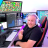# Issues with Exit Order

B

#### boogieG

##### New member
I have a basic stoch-adx strategy I'm currently backtesting that exit order is not acting correctly. I'm trying to have the exit order be triggered when price on 30m or hourly chart crosses a daily EMA. Code is below, any feedback is appreciate as I'm also not conviced the entry is totally perfect and this is one of my first scripts. Thank you in advance!
Code:
``````input over_bought = 80;
input over_sold = 20;
input KPeriod = 14;
input DPeriod = 14;
input priceH = high;
input priceL = low;
input priceC = close;
input slowing_period = 3;
input averageType = AverageType.SIMPLE;

def lowest_k = Lowest(priceL, KPeriod);
def c1 = priceC - lowest_k;
def c2 = Highest(priceH, KPeriod) - lowest_k;
def FastK = if c2 != 0 then c1 / c2 * 100 else 0;

def FullK = MovingAverage(averageType, FastK, slowing_period);
def FullD = MovingAverage(averageType, FullK, DPeriod);

def OverBought = over_bought;
def OverSold = over_sold;

def upK = FullK crosses above OverBought;
def upD = FullD crosses above OverSold;
def downK = FullK crosses below OverBought;
def downD = FullD crosses below OverBought;

input Period = AggregationPeriod.Day;
input AvgType = AverageType.EXPONENTIAL;
input Length1 = 14;
input priceclose = close;

def AVG = MovingAverage(AvgType, close(period = Period), Length1);

input length = 14;
input averageType1 = AverageType.WILDERS;

input price = close;
def sellsignal = price crosses below Avg;#### BenTen

Staff
VIP
You're trying to use multiple timeframes in the code but you did not define the hourly timeframe yet. You only have the Daily timeframe so far.

B

#### boogieG

##### New member
You're trying to use multiple timeframes in the code but you did not define the hourly timeframe yet. You only have the Daily timeframe so far.
So I would need to define hourly for the rest of the indicators?Set Position Exit to Low/High before Entry Bar? Questions 2